• 排序大体分为两类：比较排序和非比较排序一 各个排序的基本实现1.直接插入排序和希尔排序//整体思路：往一段有序区间插入元素，end标记为有序区间的最后一个元素下标，要插入的元素下标为end+1此处就称tmp，先把他...
排序大体分为两类：比较排序和非比较排序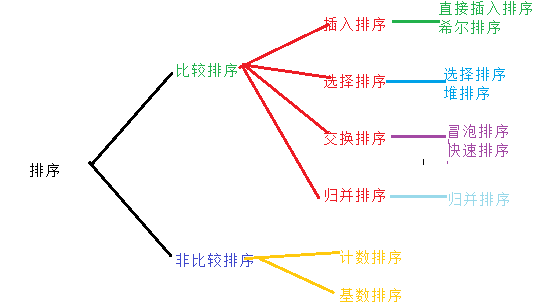一    各个排序的基本实现1.直接插入排序和希尔排序//整体思路：往一段有序区间插入元素，end标记为有序区间的最后一个元素下标，要插入的元素下标为end+1此处就称tmp，先把他保存起来，（否则可能被覆盖）如果end+1这个元素
//比有序区间的小，就把有序区间元素依次向后移动，移动结束条件有两个，1.end变为-1,2.有序区间内找到比tmp小的数。
void PrintArray ( int *a, size_t n )
{
for ( size_t i = 0; i < n ; i++ )
{
cout << a[i] << " ";
}
cout << endl;
}
//时间复杂度：o(N^2)  最坏情况，每次插入要把前面的全移动 1+2+....n-1等差数列求和(n-1)*n/2也就是o(N^2)逆序时最坏
//            o(N)    顺序最好,只比较了一遍没进去
void InsertSort ( int *a, size_t n )
{
assert ( a );

for ( size_t i = 0; i < n-1; i++ )
{
int end = i;
//单趟逻辑
int tmp = a[end + 1];
while (end>=0&& a[end] >tmp )
{
a[end + 1] = a[end];
--end;
}
a[end + 1] = tmp;
}
}
//整体思路1.预排序（使大的数很快移到后面去）分组在每组内移动接近有序 gap越大越不接近有序 2.插入排序
//1.gap>1   预排序
//2.gap==1  插入排序
void ShellSort ( int*a, size_t n )//是针对插入排序逆序的情况下，移动次数太多而设计。  希尔排序用于数据量较大
{
assert ( a );
int gap=n;
//预排序:排完说明分组为gap的这些元素各自有序
while ( gap > 1 )
{
gap = gap / 3+1;//加1保证了最后一次为gap=1，绝对会有序
for ( size_t i = 0; i<n - gap; i++ )//i++保证了不是一组走完，走另一组。而是一起走。
{
int end = i;
//单趟排序
int tmp = a[end + gap];
while ( end >= 0 && a[end] > tmp )
{
a[end+gap] = a[end ];
end -= gap;
}
a[end + gap] = tmp;
}
}
}

void TestInsertSort ( )
{
int a[] = { 2, 5, 4, 9, 3, 6, 8, 7, 1 };
//InsertSort ( a, sizeof(a) / sizeof(a));
PrintArray ( a, sizeof(a) / sizeof(a) );
}
void TestShellSort ( )
{
int a[] = { 2, 5, 4, 9, 3, 6, 8, 7, 1 };
ShellSort ( a, sizeof(a) / sizeof(a));
PrintArray ( a, sizeof(a) / sizeof(a) );
}2.选择排序和堆排序//选择排序  每次可选一个最小的数，一个最大的数
//时间复杂度  最坏o(N^2)      n-1+n-2+1  也是等差数列
//时间复杂度  最好o(N^2)     尽管你有序，可是我不知道还是要每次遍历一遍
void SelectSort ( int *a, size_t n )
{

int end = n-1;
int begin= 0;
while ( begin< end )
{
int min = begin;
int max = begin;
for ( size_t i = begin; i <= end; i++ )
{
if ( a[i]>a[max] )
{
max = i;
}
if ( a[i] < a[min ])
{
min = i;
}
}
/*	swap ( a[min], a[begin] );
if (begin== max )
{
max = min;
}
swap ( a[max], a[end] );*/
swap ( a[max], a[end] );
if ( min == end )
{
min = max;
}
swap ( a[min], a[begin] );

begin++;
end--;
}

}
//堆排序 升序 建大堆，把最大的数选出来，换到后面去，然后把剩下的数向下调整看成一个堆
//选第一个数要建堆N*lg N  其他lgn 即N*lgN+（N-1）lgN=o(NlgN)
void AdjustDown ( int *a, size_t n, int root )
{
int parent = root;
int child = 2 * parent;

while ( child<n )//如果孩子都不存在说名到叶节点，就停止向下调整
{
if ( child+1<n && a[child + 1] > a[child] )
{
child++;
}
if ( a[child] > a[parent] )
{
swap ( a[child], a[parent] );
parent = child;
child = parent * 2;
}
else
{
break;
}
}

}
void HeapSort ( int *a, size_t n )
{
for ( int i = (n - 2) / 2; i >= 0; i-- )//建堆NlgN
{
AdjustDown ( a, n, i );//lgN
}
//(N-1)lgN
int end = n - 1;
while ( end > 0 )
{
swap ( a, a[end] );
AdjustDown ( a, end, 0 );
--end;
}

}
void TestHeapSort ( )
{
int a[] = { 2, 5, 4, 9, 3, 6, 8, 7, 1, 0 };
HeapSort ( a, sizeof(a) / sizeof(a) );
PrintArray ( a, sizeof(a) / sizeof(a) );
}
void TestSelectSort ( )
{
int a[] = { 2, 5, 4, 9, 3, 6, 8, 7, 1 ,0};
SelectSort ( a, sizeof(a) / sizeof(a));
PrintArray ( a, sizeof(a) / sizeof(a) );
}
int main ( )
{
//TestSelectSort ( );
TestHeapSort ( );
system ( "pause" );
return 0;
}3.冒泡排序和快速排序冒泡排序//交换排序
//时间复杂度0(N^2) n-1+n-2+.....1  也是等差数列
//最好的情况下：0（N) 有序
//冒泡和插入的区别：插入比冒泡好，冒泡要求更严格的有序
//比如：123465  如果插入排序  是比较N-1次，插入一次 N     冒泡排序：第一趟比较 N-1次之后，已经有序可是不知道，又要来一遍 N-1
//因此插入比冒泡好，冒泡要求更严格有序

void BubbleSort ( int *a, size_t n )
{
//int end = n - 1;
//while ( end > 0 )
//{
//	bool ExChange = 0;
//	for ( size_t i = 0; i < end; i++ )//单趟
//	{
//		if ( a[i]>a[i + 1] )
//		{
//			swap ( a[i], a[i + 1] );
//			ExChange = 1;
//		}
//	}
//	if ( ExChange == 0 )
//	{
//		break;
//	}
//	end--;
//}
for ( size_t end = n - 1; end > 0; end-- )
{
bool ExChange = 0;
for ( size_t i = 0; i < end; i++ )//单趟
{
if ( a[i]>a[i + 1] )
{
swap ( a[i], a[i + 1] );
ExChange = 1;
}
}
if ( ExChange == 0 )
{
break;
}
}
}
void TestBubbleSort ( )
{
int a[] = { 2, 5, 4, 9, 3, 6, 8, 7, 1, 0 };
BubbleSort ( a, sizeof(a) / sizeof(a) );
PrintArray ( a, sizeof(a) / sizeof(a) );
}快速排序：//时间复杂度：递归的次数乘以每次递归  递归的次数N每次递归lgN   因此时间复杂度为o(NlgN)
//最坏情况：0(N^2)  有序   三数取中法，解决了有序的这种情况
int GetMidindex ( int *a, int begin, int end )
{
int mid = begin + ((end - begin) >> 1);
if ( a[mid] > a[begin] )
{
if ( a[begin] > a[end] )
{
return begin;
}
else if ( a[mid] > a[end] )
{
return end;
}
else
{
return mid;
}
}
else
{
if ( a[begin] > a[end] )
{
return begin;
}
else if ( a[end] > a[mid] )
{
return mid;
}
else
{
return end;
}
}
}
//左右指针法
int PartSort2 ( int *a, int begin, int end )
{
//int& key= a[end];//为什莫给a[end]而不是和讲的一样是a[end-1]，注意考虑有序情况：如果给a[end-1]反而错了，如果给a[end]就自己和自己交换
int mid = GetMidindex ( a, begin, end );
swap ( a[mid], a[end] );
int keyIndex = end;
int key = a[end];
while ( begin < end )
{
while ( begin<end&&a[begin] <= key )
{
begin++;
}
while (begin<end&& a[end]>=key )
{
end--;
}
swap ( a[end], a[begin] );
}
swap(a[keyIndex], a[begin]);
return begin;
}
//挖坑法
int PartSort1 ( int*a, int begin, int end )
{
int key = a[end];
while ( begin < end )
{
while (begin<end&& a[begin] <=key )
{
begin++;
}
a[end] = a[begin];
while (begin<end&& a[end] >= key )
{
end--;
}
a[begin] = a[end];
}
a[begin] = key;
return begin;
}
//前后指针法
int PartSort3 ( int *a, int begin, int end )
{
int& key = a[end];
//int key=a[end]
int cur = begin;
int prev = begin - 1;
while ( cur < end )
{
if ( a[cur] < key && (++prev) != cur )
{
swap ( a[prev], a[cur] );
}
cur++;
}
swap ( a[++prev], key );//swap(a[++prev],a[end]);
return prev;

}
void QuicksortNonR ( int *a, int left, int right )
{
stack<int >st;
st.push ( right );
st.push ( left );
while ( !st.empty ( ) )
{
int begin = st.top ( );
st.pop ( );
int end = st.top ( );
st.pop ( );
int div = PartSort3 ( a, begin, end );

if ( begin < div - 1 )
{
st.push ( div - 1 );
st.push ( begin );
}
if ( div + 1 < end )
{
st.push ( end );
st.push ( div + 1 );
}
}

}
void Quicksort ( int* a, int left,int right )
{
if ( left >= right )
{
return;
}
//小区间优化
if ( right - left < 8)//省去最后3层
{
InsertSort ( a+left, (right - left) + 1 );
return;
}
int div = PartSort3 ( a, left, right );
Quicksort ( a, left, div - 1 );
Quicksort ( a, div + 1, right );

}

//有序有两种情况：1.区间只剩一个值，说明有序
//                2.左边有序，右边有序
void TestQuickSort ( )
{
int a[] = { 5, 10, 4, 9, 20, 6, 8, 7, 1, 5 };
QuicksortNonR ( a, 0, sizeof(a) / sizeof(a) - 1 );
PrintArray ( a, sizeof(a) / sizeof(a) );
}
//int main ( )
//{
//	//TestBubbleSort ( );
//
//	TestQuickSort ( );
//	system ( "pause" );
//	return 0;
//}
4.归并排序归并排序
时间复杂度：0(NlgN)       空间复杂度：0(N)

void _MergeSort ( int *a, int left, int right, int* tmp )//tmp为什莫再外面开tmp，所有递归都可以用.如果在里面开里面每次递归都要开辟空间
{
if ( left >= right )//如果只剩一个元素，或者没有元素可以看作是有序的
{
return;
}
if ( right - left < 8 )//省去最后3层
{
InsertSort ( a + left, (right - left) + 1 );
return;
}
int div = ((right - left) >> 1) + left;
//让两段子区间有序再归并
//[left,div] [div+1 ,right]
_MergeSort ( a, left, div, tmp );
_MergeSort ( a, div + 1, right, tmp );
int index = left;
int begin1 = left; int end1 = div;
int begin2 = div + 1; int end2 = right;
while ((begin1<=end1)&&(begin2<=end2))
{

if ( a[begin1] <= a[begin2] )
{
tmp[index++] = a[begin1++];
}
else
{
tmp[index++] = a[begin2++];

}
}
while( begin1 <= end1 )
{
tmp[index++] = a[begin1++];
}
while (begin2<=end2 )
{
tmp[index++] = a[begin2++];
}
//每次归并完，再拷贝到原区间上去
index = left;
while ( index <= right )
{
a[index] = tmp[index];
++index;
}

}
void MergeSort ( int *a,size_t n)
{

int * tmp = new int[n];

_MergeSort(a, 0, n - 1,tmp);
delete[]tmp;

}
void TestMergeSort ( )
{
int a[] = { 5, 10, 4, 9, 20, 6, 8, 7, 1, 5 };
MergeSort( a,  sizeof(a) / sizeof(a));
PrintArray ( a, sizeof(a) / sizeof(a) );
}
int main ( )
{

TestMergeSort ( );
system ( "pause" );
return 0;
}5.计数排序//非比较排序
//基数排序：只能用于排整型   不多讲
//计数排序：
//直接定址法的哈希
//时间复杂度 0（max(n,range))   数据范围比较集中的时候适合用计数排序
void CountSort ( int *a, int n )
{
int max = a;
int min = a;
for ( int i = 0; i < n; i++ )
{
if ( a[i]>max )
{
max = a[i];
}
if ( a[i] < min )
{
min = a[i];
}
}
int range = max - min + 1;
int * hashtable = new int[range];//不能开n,是相对位置
memset ( hashtable, 0, sizeof(int)*range );
for ( size_t i = 0; i < n; i++ )
{
hashtable[a[i] - min]++;//   a[i]是绝对位置，记清楚此处是相对位置
}
size_t j = 0;
for ( size_t i = 0; i < range; i++ )
{

while ( hashtable[i]-- )
{
a[j] = i + min;
++j;
}
}

delete[] hashtable;
}
void TestCountSort ( )
{

int a[] = { 5, 10, 4, 9, 20, 6, 8, 7, 1, 5 };
CountSort( a,  sizeof(a) / sizeof(a));
PrintArray ( a, sizeof(a) / sizeof(a) );
}
int main ( )
{
TestCountSort ( );
system ( "pause" );
return 0;

}二   时间复杂度和空间复杂度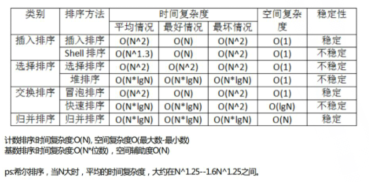三    稳定性稳定性：应用场景：成绩排名：成绩相同，先交卷子在前，后交卷子在后  具体操作：先拿时间排，再拿一个稳定的的排序对成绩排，就能保证各个排序的稳定性首先要明白所有的稳定排序都可以变成不稳定的。插入            稳定：我能做到比你小让你往后挪，和你相等放你后面如此便可以保证有序希尔            不稳定：相同的值可能被分到不同的组里面  把相对顺序打乱选择排序     不稳定 ：先选到的放到最后面取堆排序         不稳定：父亲大于等于孩子 把父亲的放到后面了，孩子的放次后面   相对位置变了冒泡            稳定 ：大的往后冒泡，相等不往后冒泡快排            不稳定：比它大的往右翻，比它小的往左翻  最后后面的那个到中间 。    // 1 9 5 7 6 4 5 8 5归并排序      稳定：如果相等时先拿左边的
展开全文• 空间复杂度 是否稳定 冒泡排序 :————-: :—–: :—–: :—–: 选择排序 :————-: :—–: :—–: :—–: 直接插入排序 :————-: :—–: :—–: :—–: 归并排序 :————-: :—–: :
排序算法分类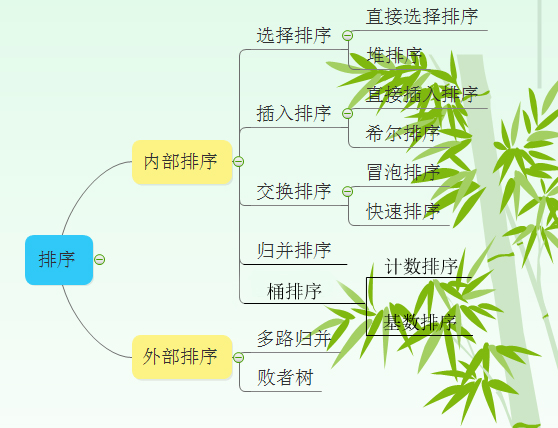排序算法比较表格填空

排序算法
平均时间复杂度
最坏时间复杂度
空间复杂度
是否稳定
冒泡排序
:————-:
:—–:
:—–:
:—–:
选择排序
:————-:
:—–:
:—–:
:—–:
直接插入排序
:————-:
:—–:
:—–:
:—–:
归并排序
:————-:
:—–:
:—–:
:—–:
快速排序
:————-:
:—–:
:—–:
:—–:
堆排序
:————-:
:—–:
:—–:
:—–:
希尔排序
:————-:
:—–:
:—–:
:—–:
计数排序
:————-:
:—–:
:—–:
:—–:
基数排序
:————-:
:—–:
:—–:
:—–:
排序算法比较表格

排序算法
平均时间复杂度
最坏时间复杂度
空间复杂度
是否稳定
冒泡排序
O（n2）<!--//--><![CDATA[//><!--
O（n^2）
//--><!]]>
O（n2）<!--//--><![CDATA[//><!--
O（n^2）
//--><!]]>
O（1）<!--//--><![CDATA[//><!--
O（1）
//--><!]]>
是
选择排序
O（n2）<!--//--><![CDATA[//><!--
O（n^2）
//--><!]]>
O（n2）<!--//--><![CDATA[//><!--
O（n^2）
//--><!]]>
O（1）<!--//--><![CDATA[//><!--
O（1）
//--><!]]>
不是
直接插入排序
O（n2）<!--//--><![CDATA[//><!--
O（n^2）
//--><!]]>
O（n2）<!--//--><![CDATA[//><!--
O（n^2）
//--><!]]>
O（1）<!--//--><![CDATA[//><!--
O（1）
//--><!]]>
是
归并排序
O(nlogn)<!--//--><![CDATA[//><!--
O(nlogn)
//--><!]]>
O(nlogn)<!--//--><![CDATA[//><!--
O(nlogn)
//--><!]]>
O（n）<!--//--><![CDATA[//><!--
O（n）
//--><!]]>
是
快速排序
O(nlogn)<!--//--><![CDATA[//><!--
O(nlogn)
//--><!]]>
O（n2）<!--//--><![CDATA[//><!--
O（n^2）
//--><!]]>
O（logn）<!--//--><![CDATA[//><!--
O（logn）
//--><!]]>
不是
堆排序
O(nlogn)<!--//--><![CDATA[//><!--
O(nlogn)
//--><!]]>
O(nlogn)<!--//--><![CDATA[//><!--
O(nlogn)
//--><!]]>
O（1）<!--//--><![CDATA[//><!--
O（1）
//--><!]]>
不是
希尔排序
O(nlogn)<!--//--><![CDATA[//><!--
O(nlogn)
//--><!]]>
O（ns）<!--//--><![CDATA[//><!--
O（n^s）
//--><!]]>
O（1）<!--//--><![CDATA[//><!--
O（1）
//--><!]]>
不是
计数排序
O(n+k)<!--//--><![CDATA[//><!--
O(n+k)
//--><!]]>
O(n+k)<!--//--><![CDATA[//><!--
O(n+k)
//--><!]]>
O(n+k)<!--//--><![CDATA[//><!--
O(n+k)
//--><!]]>
是
基数排序
O(N∗M)<!--//--><![CDATA[//><!--
O(N*M)
//--><!]]>
O(N∗M)<!--//--><![CDATA[//><!--
O(N*M)
//--><!]]>
O(M)<!--//--><![CDATA[//><!--
O(M)
//--><!]]>
是
注：

1 归并排序可以通过手摇算法将空间复杂度降到O（1），但是时间复杂度会提高。

2 基数排序时间复杂度为O（N*M），其中N为数据个数，M为数据位数。

辅助记忆

时间复杂度记忆- 冒泡、选择、直接 排序需要两个for循环，每次只关注一个元素，平均时间复杂度为O（n2）<!--//--><![CDATA[//><!--
O（n^2）
//--><!]]>（一遍找元素O(n)<!--//--><![CDATA[//><!--
O(n)
//--><!]]>，一遍找位置O(n)<!--//--><![CDATA[//><!--
O(n)
//--><!]]>）
快速、归并、希尔、堆基于二分思想，log以2为底，平均时间复杂度为O(nlogn)<!--//--><![CDATA[//><!--
O(nlogn)
//--><!]]>（一遍找元素O(n)<!--//--><![CDATA[//><!--
O(n)
//--><!]]>，一遍找位置O(logn)<!--//--><![CDATA[//><!--
O(logn)
//--><!]]>）
稳定性记忆-“快希选堆”（快牺牲稳定性） 排序算法的稳定性：排序前后相同元素的相对位置不变，则称排序算法是稳定的；否则排序算法是不稳定的。
原理理解

1 冒泡排序

1.1 过程

冒泡排序从小到大排序：一开始交换的区间为0~N-1，将第1个数和第2个数进行比较，前面大于后面，交换两个数，否则不交换。再比较第2个数和第三个数，前面大于后面，交换两个数否则不交换。依次进行，最大的数会放在数组最后的位置。然后将范围变为0~N-2，数组第二大的数会放在数组倒数第二的位置。依次进行整个交换过程，最后范围只剩一个数时数组即为有序。

1.2 动图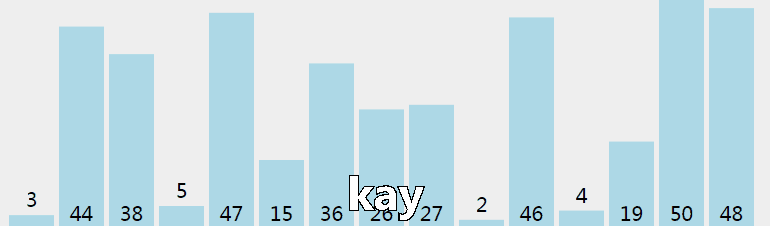1.3 核心代码（函数）

//array[]为待排序数组，n为数组长度
void BubbleSort(int array[], int n)
{
int i, j, k;
for(i=0; i<n-1; i++)
for(j=0; j<n-1-i; j++)
{
if(array[j]>array[j+1])
{
k=array[j];
array[j]=array[j+1];
array[j+1]=k;
}
}
}

2 选择排序

2.1 过程

选择排序从小到大排序：一开始从0~n-1区间上选择一个最小值，将其放在位置0上，然后在1~n-1范围上选取最小值放在位置1上。重复过程直到剩下最后一个元素，数组即为有序。

2.2 动图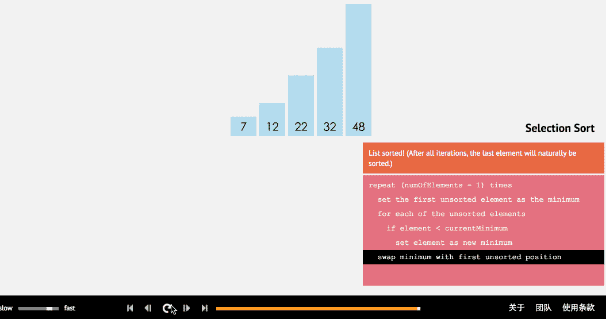2.3 核心代码（函数）

//array[]为待排序数组，n为数组长度
void selectSort(int array[], int n)
{
int i, j ,min ,k;
for( i=0; i<n-1; i++)
{
min=i; //每趟排序最小值先等于第一个数，遍历剩下的数
for( j=i+1; j<n; j++) //从i下一个数开始检查
{
if(array[min]>array[j])
{
min=j;
}
}
if(min!=i)
{
k=array[min];
array[min]=array[i];
array[i]=k;
}
}
}

3 插入排序

3.1 过程

插入排序从小到大排序：首先位置1上的数和位置0上的数进行比较，如果位置1上的数大于位置0上的数，将位置0上的数向后移一位，将1插入到0位置，否则不处理。位置k上的数和之前的数依次进行比较，如果位置K上的数更大，将之前的数向后移位，最后将位置k上的数插入不满足条件点，反之不处理。

3.2 动图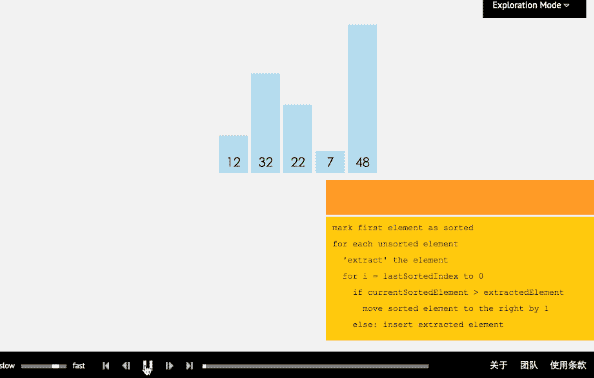3.3 核心代码（函数）

//array[]为待排序数组，n为数组长度
void insertSort(int array[], int n)
{
int i,j,temp;
for( i=1;i<n;i++)
{
if(array[i]<array[i-1])
{
temp=array[i];
for( j=i;array[j-1]>temp;j--)
{
array[j]=array[j-1];
}
array[j]=temp;
}
}
}

4 归并排序

4.1 过程

归并排序从小到大排序：首先让数组中的每一个数单独成为长度为1的区间，然后两两一组有序合并，得到长度为2的有序区间，依次进行，直到合成整个区间。

4.2 动图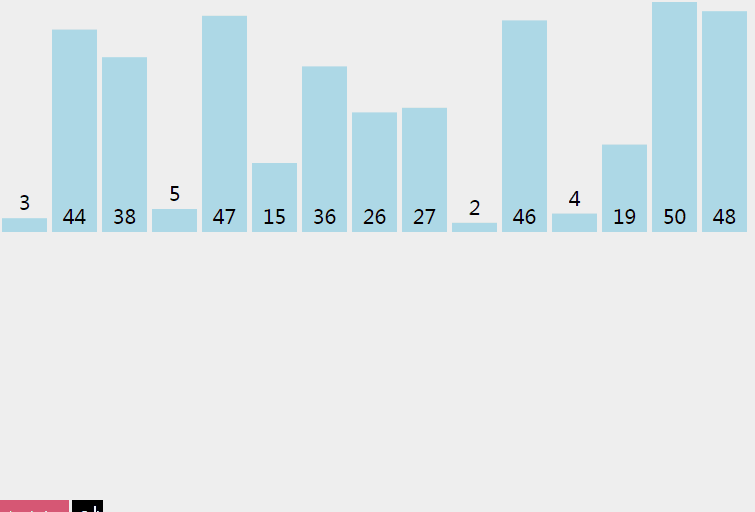4.3 核心代码（函数）

递归实现
实现归并，并把数据都放在list1里面
void merging(int *list1, int list1_size, int *list2,  int list2_size)
{
int i=0, j=0, k=0, m=0;
int temp[MAXSIZE];

while(i < list1_size && j < list2_size)
{
if(list1[i]<list2[j])
{
temp[k++] = list1[i++];
}
else
{
temp[k++] = list2[j++];
}
}
while(i<list1_size)
{
temp[k++] = list1[i++];
}
while(j<list2_size)
{
temp[k++] = list2[j++];
}

for(m=0; m < (list1_size+list2_size); m++)
{
list1[m]=temp[m];
}
}
//如果有剩下的，那么说明就是它是比前面的数组都大的，直接加入就可以了
void mergeSort(int array[], int n)
{
if(n>1)
{
int *list1 = array;
int list1_size = n/2;
int *list2 = array + n/2;
int list2_size = n-list1_size;

mergeSort(list1, list1_size);
mergeSort(list2, list2_size);

merging(list1, list1_size, list2, list2_size);
}
}
//归并排序复杂度分析：一趟归并需要将待排序列中的所有记录
//扫描一遍，因此耗费时间为O(n),而由完全二叉树的深度可知，
//整个归并排序需要进行[log2n],因此，总的时间复杂度为
//O(nlogn),而且这是归并排序算法中平均的时间性能
//空间复杂度：由于归并过程中需要与原始记录序列同样数量级的
//存储空间去存放归并结果及递归深度为log2N的栈空间，因此空间
//复杂度为O(n+logN)
//也就是说，归并排序是一种比较占内存，但却效率高且稳定的算法 

迭代实现
void MergeSort(int k[],int n)
{
int i,next,left_min,left_max,right_min,right_max;
//动态申请一个与原来数组一样大小的空间用来存储
int *temp = (int *)malloc(n * sizeof(int));
//逐级上升，第一次比较2个，第二次比较4个，第三次比较8个。。。
for(i=1; i<n; i*=2)
{
//每次都从0开始，数组的头元素开始
for(left_min=0; left_min<n-i; left_min = right_max)
{
right_min = left_max = left_min + i;
right_max = left_max + i;
//右边的下标最大值只能为n
if(right_max>n)
{
right_max = n;
}
//next是用来标志temp数组下标的，由于每次数据都有返回到K，
//故每次开始得重新置零
next = 0;
//如果左边的数据还没达到分割线且右边的数组没到达分割线，开始循环
while(left_min<left_max&&right_min<right_max)
{
if(k[left_min] < k[right_min])
{
temp[next++] = k[left_min++];
}
else
{
temp[next++] = k[right_min++];
}
}
//上面循环结束的条件有两个，如果是左边的游标尚未到达，那么需要把
//数组接回去，可能会有疑问，那如果右边的没到达呢，其实模拟一下就可以
//知道，如果右边没到达，那么说明右边的数据比较大，这时也就不用移动位置了

while(left_min < left_max)
{
//如果left_min小于left_max，说明现在左边的数据比较大
//直接把它们接到数组的min之前就行
k[--right_min] = k[--left_max];
}
while(next>0)
{
//把排好序的那部分数组返回该k
k[--right_min] = temp[--next];
}
}
}
}
//非递归的方法，避免了递归时深度为log2N的栈空间，
//空间只是用到归并临时申请的跟原来数组一样大小的空间，并且在时间性能上也有一定的提升，
//因此，使用归并排序是，尽量考虑用非递归的方法。

5 快速排序

5.1 过程

快速排序从小到大排序：在数组中随机选一个数（默认数组首个元素），数组中小于等于此数的放在左边，大于此数的放在右边，再对数组两边递归调用快速排序，重复这个过程。

5.2 动图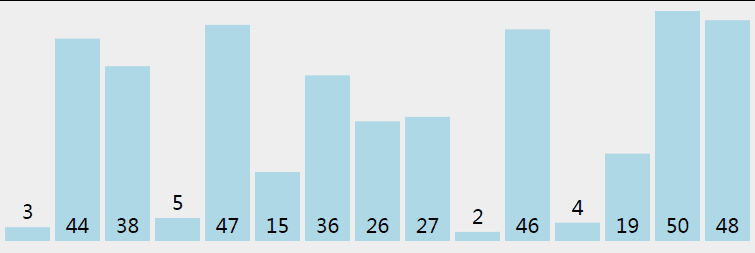5.3 核心代码（函数）

推荐程序（好理解）

//接口调整
void adjust_quicksort(int k[],int n)
{
quicksort(k,0,n-1);
}
void quicksort(int a[], int left, int right)
{
int i,j,t,temp;
if(left>right)   //（递归过程先写结束条件）
return;

temp=a[left]; //temp中存的就是基准数
i=left;
j=right;
while(i!=j)
{
//顺序很重要，要先从右边开始找（最后交换基准时换过去的数要保证比基准小，因为基准
//选取数组第一个数，在小数堆中）
while(a[j]>=temp && i<j)
j--;
//再找右边的
while(a[i]<=temp && i<j)
i++;
//交换两个数在数组中的位置
if(i<j)
{
t=a[i];
a[i]=a[j];
a[j]=t;
}
}
//最终将基准数归位 （之前已经temp=a[left]过了，交换只需要再进行两步）
a[left]=a[i];
a[i]=temp;

quicksort(left,i-1);//继续处理左边的，这里是一个递归的过程
quicksort(i+1,right);//继续处理右边的 ，这里是一个递归的过程
}  

6 堆排序

6.1 过程

堆排序从小到大排序：首先将数组元素建成大小为n的大顶堆，堆顶（数组第一个元素）是所有元素中的最大值，将堆顶元素和数组最后一个元素进行交换，再将除了最后一个数的n-1个元素建立成大顶堆，再将最大元素和数组倒数第二个元素进行交换，重复直至堆大小减为1。

注：完全二叉树
假设二叉树深度为n，除了第n层外，n-1层节点都有两个孩子，第n层节点连续从左到右。如下图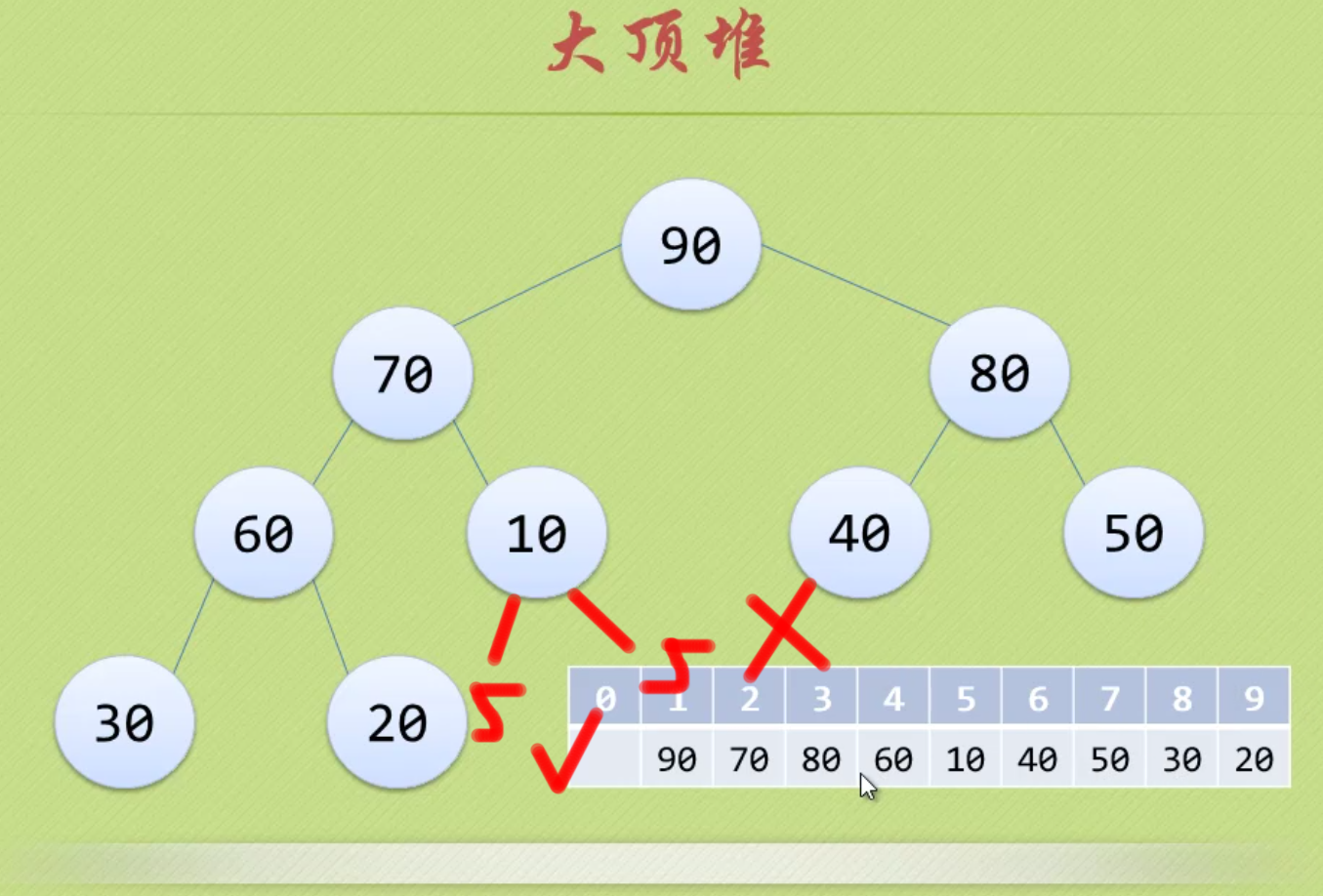注：大顶堆
大顶堆是具有以下性质的完全二叉树：每个节点的值都大于或等于其左右孩子节点的值。
即，根节点是堆中最大的值，按照层序遍历给节点从1开始编号，则节点之间满足如下关系：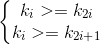(1<=i<=n/2)
6.2 动图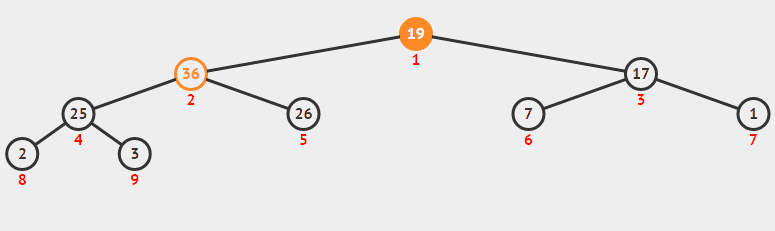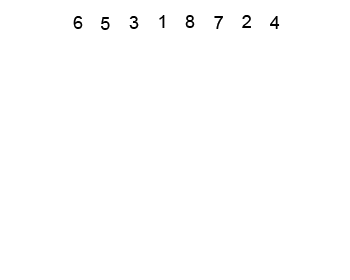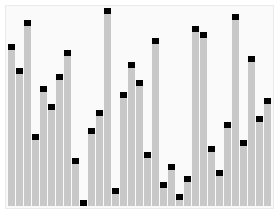6.3 核心代码（函数）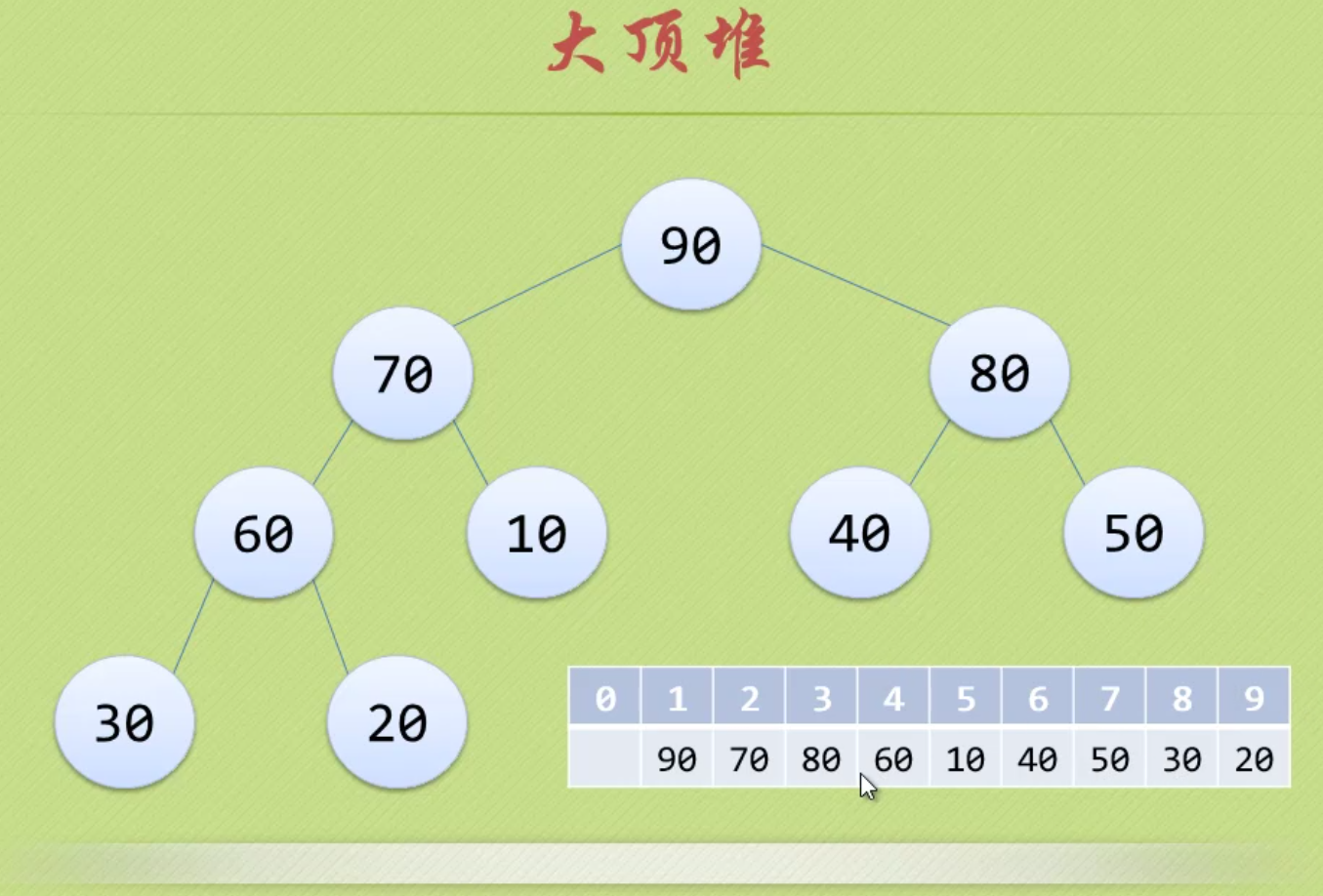注意！！！数组从1开始，1~n

void heapSort(int array[], int n)
{
int i;
for (i=n/2;i>0;i--)
{
HeapAdjust(array,i,n);//从下向上，从右向左调整
}
for( i=n;i>1;i--)
{
swap(array, 1, i);
HeapAdjust(array, 1, i-1);//从上到下，从左向右调整
}
}
void HeapAdjust(int array[], int s, int n )
{
int i,temp;
temp = array[s];
for(i=2*s;i<=n;i*=2)
{
if(i<n&&array[i]<array[i+1])
{
i++;
}
if(temp>=array[i])
{
break;
}
array[s]=array[i];
s=i;
}
array[s]=temp;
}
void swap(int array[], int i, int j)
{
int temp;

temp=array[i];
array[i]=array[j];
array[j]=temp;
}

7 希尔排序

7.1 过程

希尔排序是插入排序改良的算法，希尔排序步长从大到小调整，第一次循环后面元素逐个和前面元素按间隔步长进行比较并交换，直至步长为1，步长选择是关键。

7.2 动图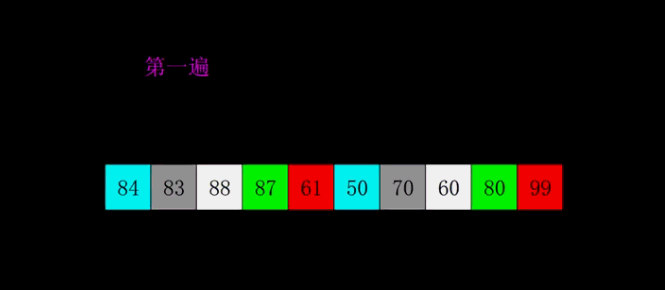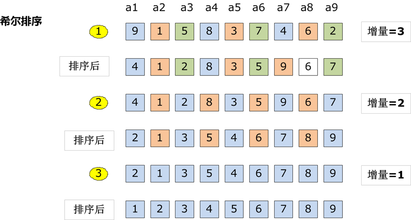7.3 核心程序（函数）

//下面是插入排序
void InsertSort( int array[], int n)
{
int i,j,temp;
for( i=0;i<n;i++ )
{
if(array[i]<array[i-1])
{
temp=array[i];
for( j=i-1;array[j]>temp;j--)
{
array[j+1]=array[j];
}
array[j+1]=temp;
}
}
}
//在插入排序基础上修改得到希尔排序
void SheelSort( int array[], int n)
{
int i,j,temp;
int gap=n; //~~~~~~~~~~~~~~~~~~~~~
do{
gap=gap/3+1;  //~~~~~~~~~~~~~~~~~~
for( i=gap;i<n;i++ )
{
if(array[i]<array[i-gap])
{
temp=array[i];
for( j=i-gap;array[j]>temp;j-=gap)
{
array[j+gap]=array[j];
}
array[j+gap]=temp;
}
}
}while(gap>1);  //~~~~~~~~~~~~~~~~~~~~~~

}

8 桶排序（基数排序和基数排序的思想）

8.1 过程

桶排序是计数排序的变种，把计数排序中相邻的m个”小桶”放到一个”大桶”中，在分完桶后，对每个桶进行排序（一般用快排），然后合并成最后的结果。

8.2 图解8.3 核心程序

#include <stdio.h>
int main()
{
int a,i,j,t;
for(i=0;i<=10;i++)
a[i]=0;  //初始化为0

for(i=1;i<=5;i++)  //循环读入5个数
{
scanf("%d",&t);  //把每一个数读到变量t中
a[t]++;  //进行计数(核心行)
}

for(i=0;i<=10;i++)  //依次判断a~a
for(j=1;j<=a[i];j++)  //出现了几次就打印几次
printf("%d ",i);

getchar();getchar();
//这里的getchar();用来暂停程序，以便查看程序输出的内容
//也可以用system("pause");等来代替
return 0;
}

9 计数排序

9.1 过程

算法的步骤如下：
- 找出待排序的数组中最大和最小的元素
- 统计数组中每个值为i的元素出现的次数，存入数组C的第i项
- 对所有的计数累加（从C中的第一个元素开始，每一项和前一项相加）
- 反向填充目标数组：将每个元素i放在新数组的第C(i)项，每放一个元素就将C(i)减去1

9.2 图解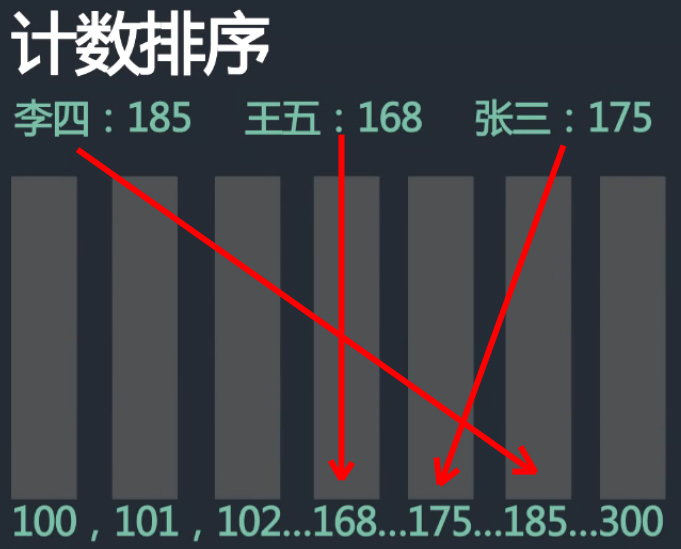9.3 核心程序（函数）

程序1：
#define NUM_RANGE (100)    //预定义数据范围上限，即K的值

void counting_sort(int *ini_arr, int *sorted_arr, int n)  //所需空间为 2*n+k
{
int *count_arr = (int *)malloc(sizeof(int) * NUM_RANGE);
int i, j, k;

//初始化统计数组元素为值为零
for(k=0; k<NUM_RANGE; k++){
count_arr[k] = 0;
}
//统计数组中，每个元素出现的次数
for(i=0; i<n; i++){
count_arr[ini_arr[i]]++;
}

//统计数组计数，每项存前N项和，这实质为排序过程
for(k=1; k<NUM_RANGE; k++){
count_arr[k] += count_arr[k-1];
}

//将计数排序结果转化为数组元素的真实排序结果
for(j=n-1 ; j>=0; j--){
int elem = ini_arr[j];          //取待排序元素
int index = count_arr[elem]-1;  //待排序元素在有序数组中的序号
sorted_arr[index] = elem;       //将待排序元素存入结果数组中
count_arr[elem]--;              //修正排序结果，其实是针对算得元素的修正
}
free(count_arr);
}

程序2：C++(最大最小压缩桶数)
public static void countSort(int[] arr) {
if (arr == null || arr.length < 2) {
return;
}
int min = arr;
int max = arr;
for (int i = 1; i < arr.length; i++) {
min = Math.min(arr[i], min);
max = Math.max(arr[i], max);
}
int[] countArr = new int[max - min + 1];
for (int i = 0; i < arr.length; i++) {
countArr[arr[i] - min]++;
}
int index = 0;
for (int i = 0; i < countArr.length; i++) {
while (countArr[i]-- > 0) {
arr[index++] = i + min;
}
}

10 基数排序

10.1 过程

基数排序是基于数据位数的一种排序算法。
它有两种算法
①LSD–Least Significant Digit first  从低位（个位）向高位排。
②MSD– Most Significant Digit first 从高位向低位（个位）排。
时间复杂度O(N*最大位数)。
空间复杂度O(N)。

10.2 图解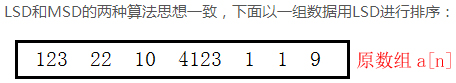对a[n]按照个位0~9进行桶排序：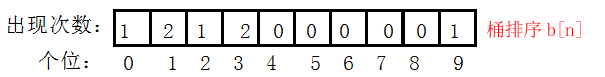对b[n]进行累加得到c[n]，用于b[n]中重复元素计数
！！！b[n]中的元素为temp中的位置！！！跳跃的用++补上：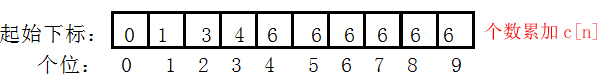temp数组为排序后的数组，写回a[n]。temp为按顺序倒出桶中的数据（联合b[n],c[n],a[n]得到），重复元素按顺序输出：10.3 核心程序

//基数排序
//LSD  先以低位排，再以高位排
//MSD  先以高位排，再以低位排
void LSDSort(int *a, int n)
{
assert(a);  //判断a是否为空，也可以a为空||n<2返回
int digit = 0;   //最大位数初始化
for (int i = 0; i < n; ++i)
{   //求最大位数
while (a[i] > (pow(10,digit)))  //pow函数要包含头文件math.h，pow(10,digit)=10^digit
{
digit++;
}
}
int flag = 1;   //位数
for (int j = 1; j <= digit; ++j)
{
//建立数组统计每个位出现数据次数（Digit[n]为桶排序b[n]）
int Digit = { 0 };
for (int i = 0; i < n; ++i)
{
Digit[(a[i] / flag)%10]++;  //flag=1时为按个位桶排序
}
//建立数组统计起始下标（BeginIndex[n]为个数累加c[n]，用于记录重复元素位置
//flag=1时，下标代表个位数值，数值代表位置，跳跃代表重复）
int BeginIndex = { 0 };
for (int i = 1; i < 10; ++i)
{
//累加个数
BeginIndex[i] = BeginIndex[i - 1] + Digit[i - 1];
}
//建立辅助空间进行排序
//下面两条可以用calloc函数实现
int *tmp = new int[n];
memset(tmp, 0, sizeof(int)*n);//初始化
//联合各数组求排序后的位置存在temp中
for (int i = 0; i < n; ++i)
{
int index = (a[i] / flag)%10;  //桶排序和位置数组中的下标
//计算temp相应位置对应a[i]中的元素，++为BeginIndex数组数值加1
//跳跃间隔用++来补，先用再++
tmp[BeginIndex[index]++] = a[i];
}
//将数据重新写回原空间
for (int i = 0; i < n; ++i)
{
a[i] = tmp[i];
}
flag = flag * 10;
delete[] tmp;
}
}  

附：

1 完整程序框架（冒泡排序举例）

1.1 VS2010程序

#include "stdafx.h"
#include "stdio.h"
#include <stdlib.h>

void BubbleSort(int array[], int n){
int i,j,k,count1=0, count2=0;
for(i=0; i<n-1; i++)
for(j=n-1; j>i; j--)
{
count1++;
if(array[j-1]>array[j])
{
count2++;
k=array[j-1];
array[j-1]=array[j];
array[j]=k;
}
}
printf("总共的循环次序为：%d,  总共的交换次序为：%d\n\n", count1, count2);
}

int main(int argc, _TCHAR* argv[])
{
int as[]={0,1,2,3,4,6,8,5,9,7};
BubbleSort(as, 10);
for(int i=0; i<10; i++)
{
printf("%d", as[i]);
}
printf("\n\n");
system("pause");
return 0;
}

1.2 执行程序（OJ）

#include <stdio.h>

void BubbleSort(int array[], int n){
int i,j,k,count1=0, count2=0;
for(i=0; i<n-1; i++)
for(j=n-1; j>i; j--)
{
count1++;
if(array[j-1]>array[j])
{
count2++;
k=array[j-1];
array[j-1]=array[j];
array[j]=k;
}
}
printf("总共的循环次序为：%d,  总共的交换次序为：%d\n\n", count1, count2);
}

int main()
{
int as[]={0,1,2,3,4,6,8,5,9,7};
BubbleSort(as, 10);
int i=0;
for(i=0; i<10; i++)
{
printf("%d", as[i]);
}
return 0;
}

2 关于交换的优化

不用中间变量进行交换

if(A[j] <= A[i]){
A[j] = A[j] + A[i];
A[i] = A[j] - A[i];
A[j] = A[j] - A[i];
}

3 C语言实现数组动态输入

#include <stdio.h>
#include <assert.h>  //断言头文件
#include <stdlib.h>

int main(int argc, char const *argv[])
{
int size = 0;
scanf("%d", &size);   //首先输入数组个数
assert(size > 0);     //判断数组个数是否非法

int *array = (int *)calloc(size, sizeof(int));  //动态分配数组
if(!R1)
{
return;           //申请空间失败
}

int i = 0;
for (i = 0; i < size; ++i) {
scanf("%d", &array[i]);
}

mergeSort(array, size);
printArray(array, size);

free(array);
return 0;
} 

注：
1.colloc与malloc类似,但是主要的区别是存储在已分配的内存空间中的值默认为0,使用malloc时,已分配的内存中可以是任意的值.
2.colloc需要两个参数,第一个是需要分配内存的变量的个数,第二个是每个变量的大小.
展开全文• 文章目录1 冒泡排序2 选择排序3 插入排序4 归并排序5 快速排序6 堆排序7 桶排序8 基数排序9 外部排序 1 冒泡排序 时间复杂度：O(n*n) 稳定性：稳定 空间复杂度：O(1) 2 选择排序 时间复杂度：O(n*n) 稳定性：不稳定 ...
文章目录1 冒泡排序2 选择排序3 插入排序4 归并排序5 快速排序6 堆排序7 桶排序8 基数排序9 外部排序
1 冒泡排序
时间复杂度：O(n*n)
稳定性：稳定
空间复杂度：O(1)
2 选择排序
时间复杂度：O(n*n)
稳定性：不稳定
空间复杂度：O(1)
3 插入排序
时间复杂度：O(n*n)
稳定性：稳定
空间复杂度：O(1)
4 归并排序
时间复杂度：O(nlogn)
稳定性：稳定
空间复杂度：O(n)
5 快速排序
时间复杂度：O(nlogn)
稳定性：不稳定
空间复杂度：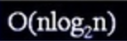最坏情况下归并排序效率大于快速排序，平均情况下两者的效率时间相同。归并排序需要的更多的额外空间。
6 堆排序
时间复杂度：O(nlogn)
稳定性：不稳定
空间复杂度：O(1)
特点：1、堆是一个完全二叉树。2、每个结点大于或者等于它的任意一个结点。
完全二叉树：除最后一层每一层都被填满，如果最后一层没有填满那么它的叶节点全部是偏向左的。
存储：如果堆的大小是已知的那么可以将堆存储在ArrayList里面，位置i处的节点左孩子是2i+1,右孩子是2i+2,父节点是(i-1)/2。
过程：将需要排序的数据全部加入到堆中，然后迭代地返回根结点。根是最大数的节点。
说明：基于比较的排序算法最好是O(nlogn)
7 桶排序
时间复杂度：O(n+N)
空间复杂度：O(n+N)
稳定性：不稳定
适用类型：小整数的排序，整数过大使用基数排序。
基于ArrayList实现
8 基数排序
时间复杂度：O(dn)基数位置的最大值。例如213基数位置的最大值是3，相当于进行了d次桶排序。
稳定性：稳定
空间复杂度：O(dn)
特点：使用十个桶，桶排序是稳定的。
9 外部排序


展开全文• 其实选择排序是非常简单的，和冒泡排序有异曲同工之妙。就是把元素分成两部分，一部分是有序的，另外一部分是无序的；每次循环从无序的元素中选取一个元素放到有序的元素中，依次循环到最后把所有元素都放到了有序那...
        选择排序（Selection sort）是一种简单直观的排序算法。它的工作原理是每一次从待排序的数据元素中选出最小（或最大）的一个元素，存放在序列的起始位置，直到全部待排序的数据元素排完。 选择排序是不稳定的排序方法（比如序列[5， 5， 3]第一次就将第一个与交换，导致第一个5挪动到第二个5后面）

算法分析
其实选择排序是非常简单的，和冒泡排序有异曲同工之妙。就是把元素分成两部分，一部分是有序的，另外一部分是无序的；每次循环从无序的元素中选取一个元素放到有序的元素中，依次循环到最后把所有元素都放到了有序那一部分中（也就是无序部分，元素为零）；        基本思路：
1、外循环：循环每个位置（其实就是选择了这个位置，然后用内循环去选择一个合适的数，放到这个位置）；
2、内循环：在无序元素中选择一个合适的数；
3、把第二步选中的数据放到第一步选中的位置上就可以了；

实现代码

#include<stdio.h>
// 打印数组元素
void print_array(int *array, int length)
{
int index = 0;
printf("array:\n");
for(; index < length; index++){
printf(" %d,", *(array+index));
}
printf("\n\n");
}

void selectSort(int array[], int length)
{
int i, j, tmp;
// 条件判断
if (1 >= length) return;
// 循环每个位置，为该位置选择合适数据
for (i = 0; i < length; i++){
tmp = i;
for (j = i; j < length; j++){
if (array[tmp] < array[j]) tmp = j;// 选择合适数据
}
if (i != tmp){   // 把选择好的数据放到外循环中选中的位置中
j = array[tmp];
array[tmp] = array[i];
array[i] = j;
}
}
}

int main(void)
{
int array = {1,11,12,4,2,6,9,0,3,7,8,2};
print_array(array, 12);
selectSort(array, 12);
print_array(array, 12);
return 0;
}

运行结果：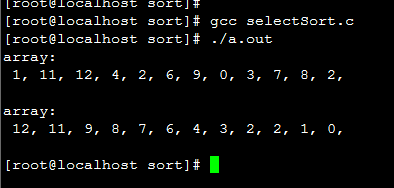时间复杂度
选择排序的时间复杂度不像前面几种排序方法那样，前面几种排序方法的时间复杂度不是一眼就能看出来的，而是要通过推导计算才能得到的。一般会涉及到递归和完全二叉树，所以推导也不是那么容易。但是选择排序就不一样了，你可以很直观的看出选择排序的时间复杂度：就是两个循环消耗的时间；
比较时间：T = （n-1)）+ （n -2）+（n - 3）.... + 1;  ===>>  T =  [n*(n-1) ] / 2；
交换时间：最好的情况全部元素已经有序，则 交换次数为0；最差的情况，全部元素逆序，就要交换 n-1 次；
所以最优的时间复杂度  和最差的时间复杂度   和平均时间复杂度  都为 ：O(n^2)

空间复杂度
空间复杂度，最优的情况下（已经有顺序）复杂度为：O(0) ；最差的情况下（全部元素都要重新排序）复杂度为：O(n );；平均的时间复杂度：O(1)

转载请注明作者和原文出处，原文地址：http://blog.csdn.net/yuzhihui_no1/article/details/44339673
若有不正确之处，望大家指正，共同学习！谢谢！！！


展开全文排序算法 冒泡排序
• 1.选取主元选取一个元素为主元，这里采用三个数(left, center, right)的中位数作为主元的方法，并把选择好的主元放到right-1的位置(升序排序)，这样接下来的子集划分只需要从left+1，right-2开始即可 2.子集划分...
• 四大排序的时间复杂度和空间复杂度时间复杂度时间复杂度的表示方法时间复杂度的分析和计算方法常见的几种时间复杂度常见的时间复杂度排序空间复杂度 时间复杂度 (1)时间频率 一个算法执行所耗费的时间，从理论上是不...
• 快速排序也不是万能的，例如当给定序列规模很小时，选择排序/插入排序就要比快排好很多。 优：快,空间占用小 缺：不稳定 适用：是目前基于比较的内部排序中被认为是最好的方法，当待排序的关键字是随机分布时，不...
• 直接上代码，看注释就能懂 时间复杂度： ...空间复杂度：O(1)。 不稳定 package sort; public class SimpleSelectSort { public static void simpleSelectSort(int array[]) { int temp = array[...
• 选择排序空间复杂度：O（1） 插入排序的时间复杂度：O（n^2） 插入排序的空间复杂度：O（1） 希尔排序的时间复杂度：O（n^（3/2）） 希尔排序的空间复杂度：O（1） 归并排序的时间复杂度：O（n*logn） 归并排序的...算法 c语言
• 时间复杂度和空间复杂度的简述 时间复杂度 **定义：**每个算法都有自己的执行时间，但我们无法算出来，只能上机去测试。但不是所有的算法都去上机测试，我们只需要知道那个算法的循环次数少也就知道这个算法执行时间...c++ 数据结构 算法
• 常用排序算法时间复杂度、空间复杂度总结。包括：冒泡排序、快速排序、选择排序、堆排序、插入排序、Shell排序、归并排序、基数排序。
• 排序方法 平均时间复杂度 最好 最差 空间复杂度 稳定度 冒泡排序 o(n^2) o(n) o(n^2) o(1) 稳定 选择排序 o(n^2) ...时间复杂度
• 空间复杂度 时间复杂度（平均/最坏） 稳定性 冒泡排序 O(1) O(n2)/O(n2) 稳定 选择排序 O(1) O(n2)/O(n2) 不稳定 插入排序 O(1) O(n2)/O(n2) 稳定 希尔排序 O(1) O(nlogn)/O(ns) （s为步长） 不稳定 ...
• 1 归并排序可以通过手摇算法将空间复杂度降到O（1），但是时间复杂度会提高。 2 基数排序时间复杂度为O（N*M），其中N为数据个数，M为数据位数。 辅助记忆 时间复杂度记忆- 冒泡、选择、直接 排序需要两个for...
• 空间复杂度 稳定性 选择排序 Selection n2 n2 n2 1 不稳 冒泡排序 Bubble ...
• 空间复杂度 稳定性 冒泡排序 O(n²) O(n²) O(n) O(1) 稳定 直接选择排序 O(n²) O(n²) ...
• 八大排序算法时间复杂度和空间复杂度对比 排序有内部排序和外部排序，内部排序是数据记录在内存中进行排序，而外部排序是因排序的数据很大，一次不能容纳全部的排序记录，在排序过程中需要访问外存。 八大排序就是...java 数据结构
• 各种排序算法时间复杂度和空间复杂度表: 比较时间复杂度函数的情况如下图： 对n较大的排序记录。一般的选择都是时间复杂度为O(nlog2n)的排序方法： 接下来博主抽时间要整理一下各经典算法思想和心得，敬请期待算法
• 常见的排序算法有：内部排序和外部排序算法，我们所接触到的八大排序算法都属于内部排序。...它们的时间复杂度空间复杂幅度分别是： 在基数排序中，r代表关键字的基数，d代表关键字的长度，n代表关...算法
• 以上是“比较排序”方法，这里我把冒泡排序、简单选择排序、直接插入排序称为简单排序。 那么，可做以下记忆： 平均时间复杂度：简单排序为，其他为，希尔排序略有区别。 稳定性：洗牌快洗对，不稳定。快速排序、...
• ## 排序算法复杂度

千次阅读 2020-07-18 11:46:19
简单选择排序 O(n2) O(n2) O(n2) O(1) 稳定 直接插入排序 O(n2) O(n) O(n2) O(1) 稳定 希尔排序 O(nlogn) O(n1.3) O(n2) O(1) 不稳定 堆排序 O(nlogn)) O(nlogn) O(nlogn) O(1) 不稳定 归并排序 O(nlogn)) O...算法
• 排序（Sorting）问题是我们再业务开发中遇到的最基本问题，因此成为各大IT公司招聘笔试面试必考内容之一。笔者也借着校园招聘对...选择排序直接选择排序 堆排序 归并排序 排序时间复杂度和空间复杂度 类别 平均时间java 数据结构
• 常用的排序算法的时间复杂度和空间复杂度 排序法 最差时间分析 平均时间复杂度 稳定度 空间复杂度 冒泡排序 O(n2) O(n2) 稳定 O(1) 快速排序 O(n2) O(nlog2n) 不稳定 O(log2n)~O(n) 选择排序 O(n2)
• 额外空间复杂度 稳定性 简单选择排序 O(N2) O(N2) O(1) 不稳定 冒泡排序 O(N2) O(N2) O(1) 稳定 直接插入排序 O(N2) O(N2) O(1) 稳定 希尔排序 O(Nd) O(N2) O(1) 不稳定 堆排序 O(NlogN) O(NlogN) O(1) ...
• 一、排序算法的时间复杂度及空间复杂度 冒泡： 平均O(N2) ,最坏O(N2) ，最好O(N) ，辅助内存O(1),稳定排序 最好情况是加了改进方法的最好：即冒泡的过程中检查是否发生了交换，如果没有发生交换，说明都排好序了...
• 时间复杂度： 比较 第一次从0到n-1中选出最小的数，与A交换位置 第二次从1到n-1中选出最小的数，与A...空间复杂度： 最好，不交换，不用临时变量，O(0) 最坏，每次交换，用n-1个临时变量，O(n) 平均，O(1) ...java...